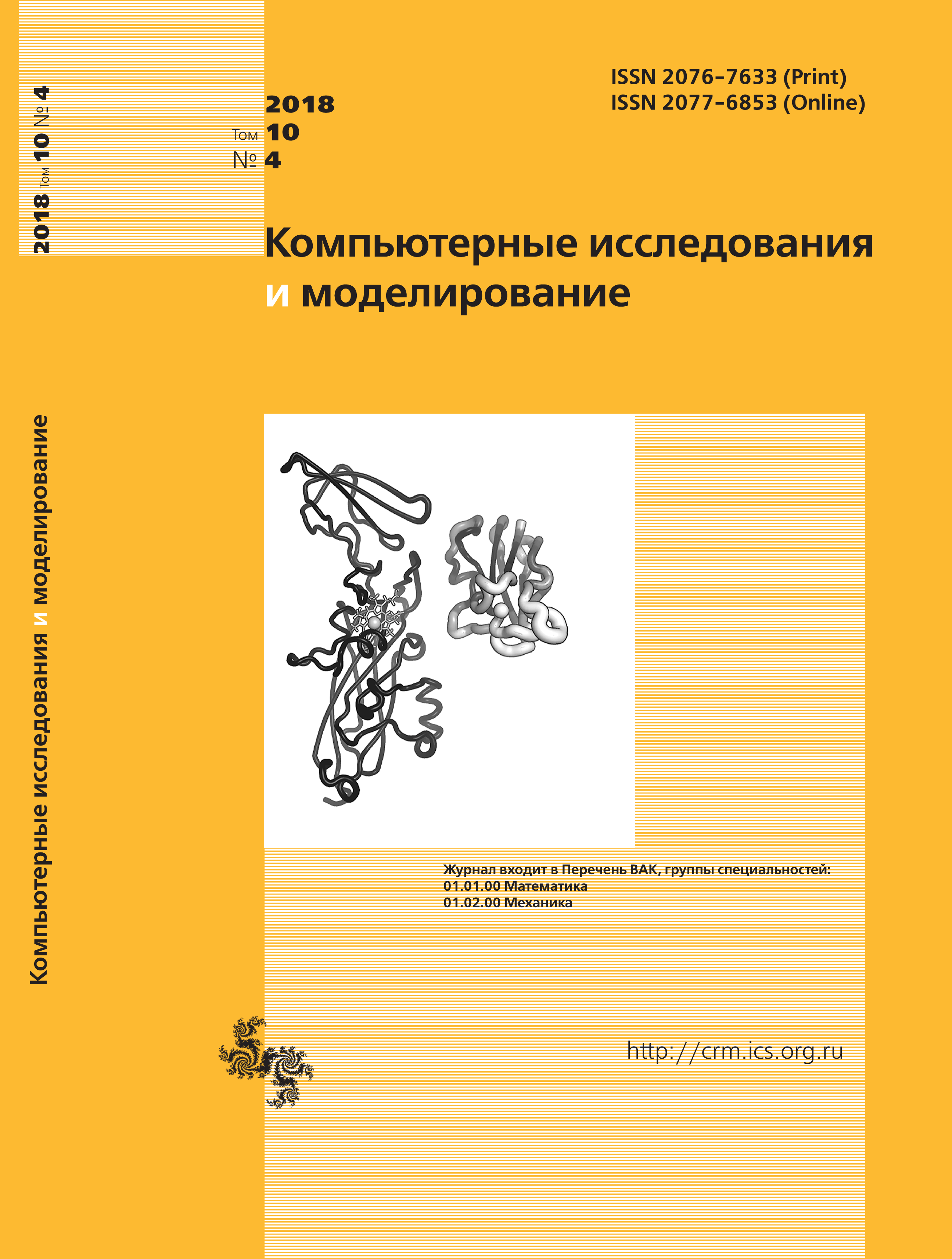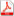Number 4, 2018 Vol. 10

All issues

Determination of CT dose by means of noise analysispdf (8789K)  / List of references

The article deals with the process of creating an effective algorithm for determining the amount of emitted quanta from an X-ray tube in computer tomography (CT) studies. An analysis of domestic and foreign literature showed that most of the work in the field of radiometry and radiography takes the tabulated values of X-ray absorption coefficients into account, while individual dose factors are not taken into account at all since many studies are lacking the Dose Report. Instead, an average value is used to simplify the calculation of statistics. In this regard, it was decided to develop a method to detect the amount of ionizing quanta by analyzing the noise of CT data. As the basis of the algorithm, we used Poisson and Gauss distribution mathematical model of owns’ design of logarithmic value. The resulting mathematical model was tested on the CT data of a calibration phantom consisting of three plastic cylinders filled with water, the X-ray absorption coefficient of which is known from the table values. The data were obtained from several CT devices from different manufacturers (Siemens, Toshiba, GE, Phillips). The developed algorithm made it possible to calculate the number of emitted X-ray quanta per unit time. These data, taking into account the noise level and the radiuses of the cylinders, were converted to X-ray absorption values, after which a comparison was made with tabulated values. As a result of this operation, the algorithm used with CT data of various configurations, experimental data were obtained, consistent with the theoretical part and the mathematical model. The results showed good accuracy of the algorithm and mathematical apparatus, which shows reliability of the obtained data. This mathematical model is already used in the noise reduction program of the CT of own design, where it participates as a method of creating a dynamic threshold of noise reduction. At the moment, the algorithm is being processed to work with real data from computer tomography of patients.

Keywords: computed tomography, radiation dose, CT noise level, X-ray attenuation coefficient
Citation in English: Usanov M.S., Kulberg N.S., Yakovleva T.V., Morozov S.P. Determination of CT dose by means of noise analysis // Computer Research and Modeling, 2018, vol. 10, no. 4, pp. 525-533
DOI: 10.20537/2076-7633-2018-10-4-525-533

Full-text version of the journal is also available on the web site of the scientific electronic library eLIBRARY.RU

The journal is included in the Russian Science Citation Index

The journal is included in the List of Russian peer-reviewed journals publishing the main research results of PhD and doctoral dissertations.

International Interdisciplinary Conference "Mathematics. Computing. Education"

The journal is included in the RSCI

Indexed in Scopus